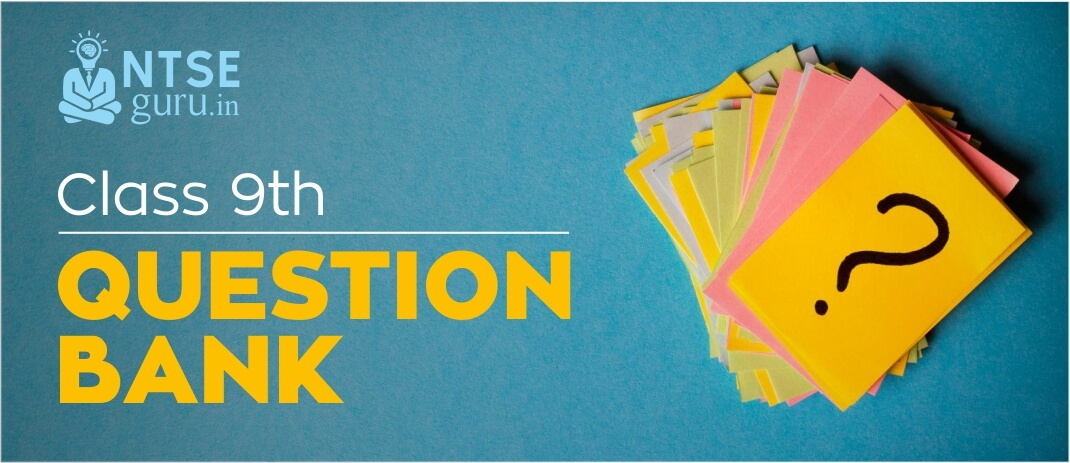# Question Bank for Class 9 CBSEExam preparation can only be tested using tools like question bank. These are the source of all the important questions compiled together to provide you with easier access and yet boost your preparation as well as confidence.

If you are at your last-minute preparation and want something to test your preparation of all the subjects, then carry on with the question bank for class 9 CBSE given below.

## 9th Class Question Bank

• 1 The polynomial ax³ + 3x² - 3 and 2x³ - 5x + a leave the same remainder when divided by x-4. find the value of a.

• 2. Represent √5.5 on the number line. Find four rational numbers between 1/2 and 1/3.

• 3. Define the following terms:
(i) Line segment (ii) Collinear points (iii) Parallel lines (iv) Intersecting lines (v) Concurrent lines (vi) Ray

• 4. Prove that "angles opposite to equal sides of an isosceles triangle are equal".

• 5. Two parallel chords of lengths 30 cm and 16 cm are drawn on the opposite sides of the centre of circle of radius 17 cm. Find the distance between the chords.

• 6. A body can have zero average velocity but not zero average speed. Justify giving an example.

• 7. (a) State Newton's first law of motion.
(b) which law is also called as 'law of inertia'?
(c) Which is correct statement?
(i) A force is required to change the state of an object from rest to motion.
(ii) An unbalanced force is required to change the state of rest.

• 8. Define boiling point. Write down the boiling point of water in Celsius scale and Kelvin scale.

• 9. a solution is prepared by adding 40 g of sugar in 100 g of water. Calculate the concentration in terms of mass by mass percentage of solution.

• 10. How will you separate a mixture of ammonium chloride, sodium chloride and Sand?

• 11. State the function of chromosomes in a cell.

• 12. Differentiate meristematic tissues from permanent tissues.

• 13. What do you mean by open circulatory system? Name two phylums where organism shows open circulatory system.

• 14. What was the immediate cause of French Revolution?

• 15. How did the Bolsheviks organize the October Revolution?

• 16. What is the difference between a Gorge and a Rift valley?

• 17. What are Jet Streams?

• 18. Modern farming methods require more inputs which are manufactured in industry. Do you agree?

• 19. What are the issues related to poverty which in turn become its causes?

• 20. What are the two important features of democracy?

• 21. What is the importance of the constitution?

If this question bank for class 9 CBSE has proved useful to you then you can access the full-length version with chapter-wise important questions by logging on NTSEGuru or by downloading free NTSEGuru mobile app for android.

## Related PostsHelpline No.: 9174408561